## @Smerity

### Recent Past

• Natural Language Processing (field of Machine Learning) @ Sydney University
• First Class Honours with University Medal
• Analytics & Data Mining @ Freelancer.com

### Present

• Consulting on machine learning & data mining for companies & start-ups

## Why build a robotic army?

Each and every one of us have tried taking over the world.

Admit it.

What's the fundamental problem?

Low quality henchmen & henchwomen.

Why not replace your henchpeople with cheap, expendable and scary looking robots?

## Machine Learning is scary

http://thekeyofe.deviantart.com/art/Gir-Duty-Mode-255737617

## Machine Learning is scary

• Logistic Regression
• Support Vector Machines
• Maximum Entropy classifiers
• Stochastic Gradient Descent
• Random Forest classifiers
• Latent Dirichlet Allocation
• Neural Networks

## Machine Learning is not scary

• Logistic Regression
• Support Vector Machines
• Maximum Entropy classifiers
• Stochastic Gradient Descent
• Random Forest classifiers
• Latent Dirichlet Allocation
• Neural Networks

• For the most part, Machine Learning is conceptually simple
• For the genuinely scary parts, the concepts are already implemented by Smart People™
• Crash course in Machine Learning using prior knowledge of TicTacToe

## What is machine learning (ML)?

• Allow a computer to learn, with experience, how to accurately predict a value for something previously unseen
• Is that a moon or a space station?
• Should we take off and nuke the entire site from orbit?
• What's the probability the princess is in another castle?

• Given training data with features X and a target value or label Y,
accurately predict Y' when only given the (likely unseen) features X'

## What is machine learning (ML)?

### Regression

Given our features X, we want to predict a numeric value Y

400 people walk into my store, 70% are male, 20% have iPhones, ...:
how many sales am I likely to make?

### Classification

Given our features X, we want to set Y to the class it belongs to
(i.e. is it [Mac, Linux, Windows] | [C3P0, R2D2] | [dead, alive, Schrödinger's cat])

Error is usually calculated by...

• Precision: I predicted 10 of you in here are Terminators, what percentage of you are actually Terminators?
• Recall: There were 20 Terminators in here, what percentage did I find?

## Lines: Linear Regression (regression)

• Target: a numeric value
• Aiming to: reduce the error (or distance to the line)
• Each feature xk in X has a weight λk that's learned
• If many people walk into the store, that contributes positively to the number of sales
• The target_value is computed by λ0 + x1λ1 + x2λ2 + ...
•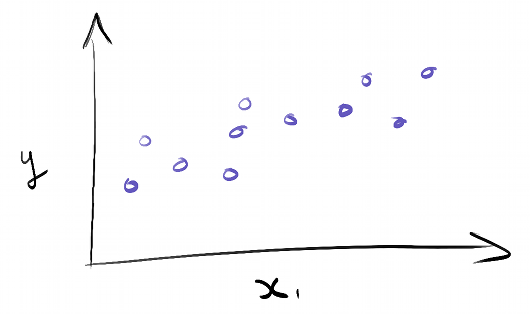## Lines: Linear Regression (regression)

• Target: a numeric value
• Aiming to: reduce the error (or distance to the line)
• Each feature xk in X has a weight λk that's learned
• If many people walk into the store, that contributes positively to the number of sales
• The target_value is computed by λ0 + x1λ1 + x2λ2 + ...
•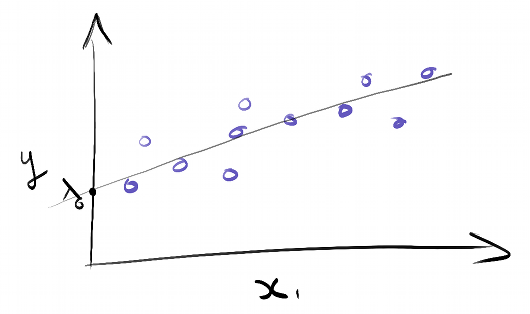## Lines: Linear Regression (regression)

• Target: a numeric value
• Aiming to: reduce the error (or distance to the line)
• Each feature xk in X has a weight λk that's learned
• If many people walk into the store, that contributes positively to the number of sales
• The target_value is computed by λ0 + x1λ1 + x2λ2 + ...
•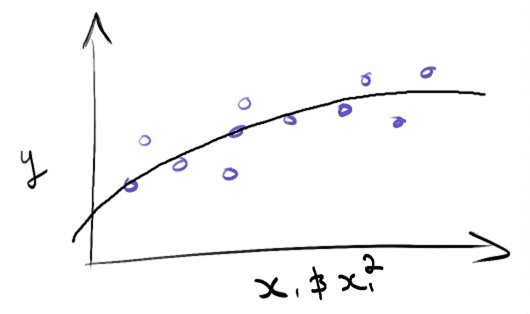## Lines: Linear Regression (regression)

• Target: a numeric value
• Aiming to: reduce the error (or distance to the line)
• Each feature xk in X has a weight λk that's learned
• If many people walk into the store, that contributes positively to the number of sales
• The target_value is computed by λ0 + x1λ1 + x2λ2 + ...
•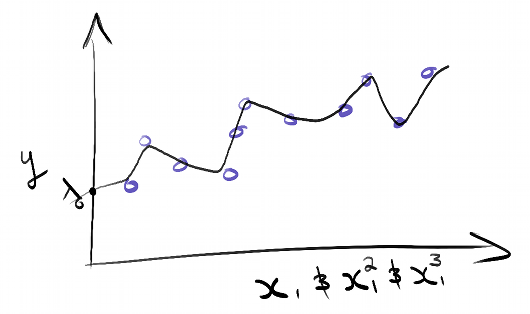## Example: Stealing Jet Fighters for Fun & Profit

For our DastardlyPlan™, we need jet fighters... Lots of jet fighters.
It turns out the airforce doesn't like publishing where they keep their fighters?

Many Bothan spies died bringing us this training data...
We need to be able to predict the # of jet fighters with easily obtained features!

Target = predict field #11, the total number of jet fighters at an airbase

Features = [ #2=area of airbase, #3=area of the runways, #7=age of base, etc. ]

```# 1      2      3      4    5  6   7  8  9  10 11
1.0   3.4720  0.998   1.0   7  4  42  3  1  0  fighters=25
1.0   3.5310  1.500   2.0   7  4  62  1  1  0  fighters=29
1.0   9.5200  1.501   0.0   6  3  32  1  1  0  fighters=28
2.5   9.8000  3.420   2.0  10  5  42  2  1  1  fighters=84
2.5  12.8000  3.000   2.0   9  5  14  4  1  1  fighters=82
...
```

## Linear Regression with Scikit-Learn

```pip install scikit-learn
```
```from sklearn.linear_model import LinearRegression
from sklearn.cross_validation import train_test_split

# Get your dataset and split it into training and testing
target_values, features = get_data()
# target_values is just an array with the number of anti-robotics specialists
# features is just an array with the feature values
train_feats, test_feats, train_values, test_values = train_test_split(feats, target_values, test_size=0.20)

regr = LinearRegression()
# This is the training step where the algorithm learns the weights to associate with each feature
regr.fit(train_feats, train_values)
predicted_values = regr.predict(test_feats)
```

## Avoiding Overfitting

#### "I studied really hard and memorised all the answers to the multiple choice!"

If you let them, most ML algorithms will "memorise" their training data.

This is sort of like memorising the answers to the practice multiple choice for use in the exam's multiple choice. Generally not a good idea!

### ℓ2 Regularisation

Penalise any large weights strongly (i.e. don't rely on one feature too much)

### ℓ1 Regularisation

Penalises large weights and encourages weights to zero (i.e. use few features)

Both of these take a value α to decide how much regularisation should occur.

## Avoiding Overfitting

#### "I studied really hard and memorised all the answers to the multiple choice!"

Other than selecting alpha, Scikit-Learn makes regularisation simple too!

```from sklearn import linear_model
regr = linear_model.LinearRegression()

alpha = α = ... # How strong should the regularization be?

# Ridge regression is linear regression with L2 regularization (i.e. encourage weights to not be large)
regr = linear_model.Ridge(alpha=alpha)

# Lasso regression is linear regression with L1 regularization (encourage features to be small and zero)
regr = linear_model.Lasso(alpha=alpha)
```

## Cross Validation

#### Hack, slash, and permute your way to victory!

Training and testing on the same data leads to overfitting.

Better idea: Train & test on different subsets of the data!

K-fold: Split data into N parts, use N-1 for training and 1 for testing.
Average out the N results.

Excellent way to select parameters (such as alpha) without overfitting

```from sklearn import cross_validation
# Ten fold cross validation has been shown to avoid overfitting in most cases
kf = cross_validation.KFold(len(data), k=10)

for train_index, test_index in kf:
train_feats, test_feats = data[train_index], data[test_index]
```

## Cross Validation for the Rushed and/or Lazy

Scikit-Learn likes you so much it even lets you cheat.

Ridge, Lasso and many others have algorithms with cross validation built-in:
see RidgeCV & LassoCV for example

The algorithm already knows what parameters it needs tuned and handles it itself!

```# No more alpha to worry about, just...
regr = linear_model.RidgeCV()
# or
regr = linear_model.LassoCV()
```

## What does regularisation look like?

Testing the different regressors over a really small dataset, we can see the impact that regularisation has.
(if you're following along at home, press H to highlight)

```Testing the LinearRegression regressor
Weights = λ = +0.5515 | +14.2133 | -4.1972 | -0.5180 | +1.4905
Average distance predicted value is from real value: 8.29
=-=-=-=-=-=-=-=-
Testing the RidgeCV regressor (L2 => avoid relying on any one feature too much)
Weights = λ = +0.9393 | +09.1384 | -0.1471 | -0.5038 | +2.7891
Average distance predicted value is from real value: 7.44
=-=-=-=-=-=-=-=-
Testing the LassoCV regressor (L1 => encourage using few features)
Weights = λ = +0.0000 | +09.1478 | +0.0000 | -0.4835 | +0.8389
Average distance predicted value is from real value: 7.64
```

## Classification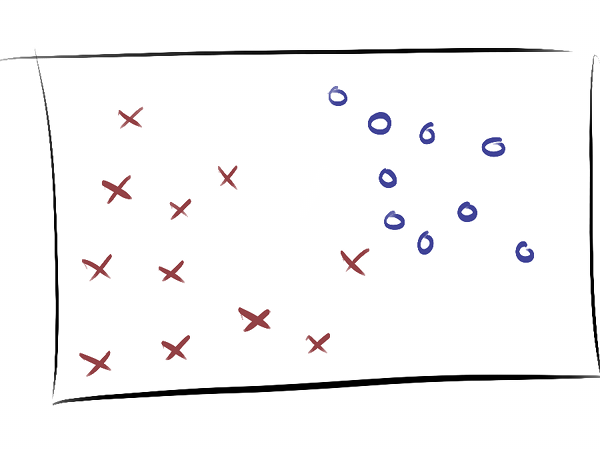## Lines: Support Vector Machines (classification)

• Target: binary classification
• Aiming to: find the widest margin between the 'good' & 'bad' sides of town
• Directly aims to maximise accuracy by not focusing on probabilties
•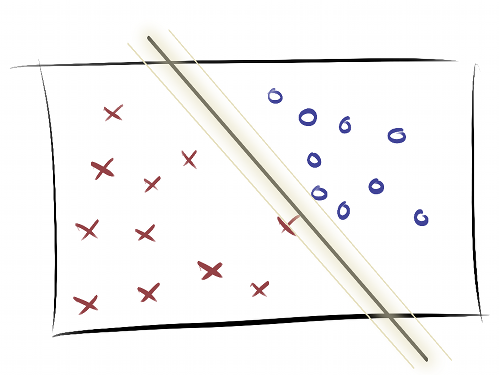## Lines: Logistic Regression (classification)

• Target: the probability Y is true given we've seen the features X or P(Y=1|X)
• Threshold Target: binary classification (Y=1 if P(Y=1|X) >= 0.5, else Y=0)

• Aiming to: work out the odds ratio of each feature xk
• Given a 19 year old female passenger on the Titanic, how much more (or less) likely is she to survive if she's ten years older?
• How does the probability of Y being true change as we increase the feature xk?
•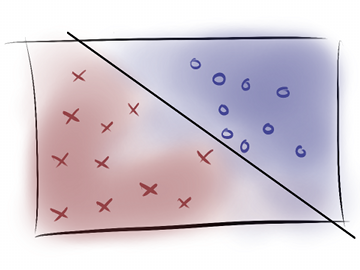## Decision Trees

### The Biggest Spaghetti Code Nested If Block You've EVER Seen!

```If person is made of metal, Android
Else
If glowing red eyes, Android
Else, Human
```## Random Forests

### Lots of a dumb thing must be good... Right?

• Creates N decision trees from different feature & training data subsets
• Decision is the average of all the N decision trees

• Avoid overfitting easily vs raw decision trees
• Commonly achieve competitive results "out of the box"## What do the classifiers look like?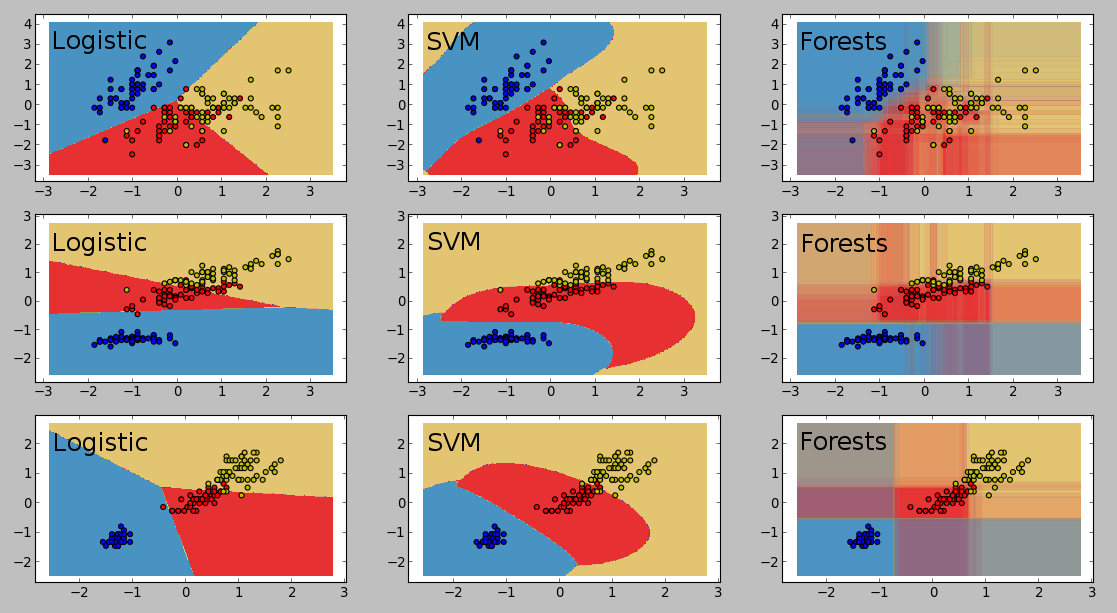## What about non-linear problems?

• Logistic regression and linear regression don't like them, at all..!
• SVM can do a magic trick and handle it easily
• SVM "lays the points on a hill" using kernel functions
• Decision trees / random forests handle it trivially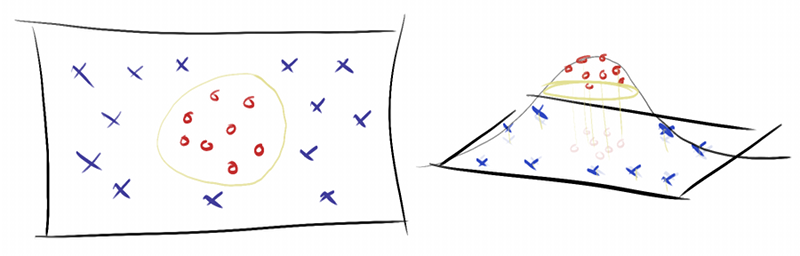## So, if I'm not taking over the world...

### Regression

• Predict & optimise for revenue
• Predict traffic to website based on holidays, weekly trends, ...
• Predict how many stars a review would get on a website

### Classification

• Classify an entry/comment/product review as fake or spam
• Predict which programming language is a user's favourite
• Automatically tag blog posts/articles/websites based upon content

### Fun!

• Explore ML on Kaggle.com -- current introductory competition teaches you to avoid overfitting using a 'who survived the the Titanic' dataset

## Questions?

### I look forward to your kind & benevolent dictatorship!

Website: www.smerity.com

Twitter: @Smerity

Email: smerity@smerity.com